Courses

# NCERT Solutions(Part- 3)- Linear Equations in One Variable Class 8 Notes | EduRev

## Class 8 Mathematics by VP Classes

Created by: Vp Classes

## Class 8 : NCERT Solutions(Part- 3)- Linear Equations in One Variable Class 8 Notes | EduRev

The document NCERT Solutions(Part- 3)- Linear Equations in One Variable Class 8 Notes | EduRev is a part of the Class 8 Course Class 8 Mathematics by VP Classes.
All you need of Class 8 at this link: Class 8

EXERCISE 2.3

Ques: Solve the following equations and check your results.
1. 3x = 2x + 18
2. 5t – 3 = 3t – 5
3. 5x + 9 = 5 + 3x
4. 4z + 3 = 6 + 2z
5. 2x – 1 = 14 – x
6. 8x + 4 = 3(x – 1) + 7
7.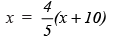8.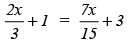9.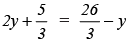10. 3m = 5m - 8/5

Ans:
1. 3x = 2x + 18
Transposing 2x from RHS to LHS, we have

3x – 2x = 18
x = 18

2. 5t – 3 = 3t – 5
Transposing (–3) to RHS, we have
5t = 3t – 5 + 3
5t = 3t – 2
Transposing 3t to LHS, we have
5t – 3t = –2
2t = –2
Diving both sides by 2, we have
t = –1

3. 5x + 9 = 5 + 3x

Transposing 9 to RHS, we have
5x = 5 + 3x – 9
5x = –4 + 3x
Transposing 3x to LHS, we have
5x – 3x = –4
2x = –4
Dividing both sides by 2, we have
x = -4/2 = -2
x =  -2

4. 4z + 3 = 6 + 2z

Transposing 3 to RHS, we have

4z = 6 – 3 + 2z
4z = 3 + 2z

Transposing 2z to LHS, we have

4z – 2z = 3
2z = 3

Dividing both sides by 2, we have
z = 3/2

5. 2x – 1 = 14 – x

Transposing (–1) to RHS, we have

2x = 14 – x + 1
2x = 15 – x

Transposing (–x) to LHS, we have
2x + x = 15
3x = 15
Dividing both sides by 3, we have

x = 15/3 = 5
∴ x = 5

6.  8x + 4 = 3(x – 1) + 7
8x + 4 = 3x – 3 + 7
8x + 4 = 3x + 4
Transposing 4 to RHS, we have
8x = 3x + 4 – 4
Transposing 3x to LHS, we have
8x – 3x = 0
5x = 0
∴  x = 0

7.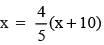Using distributive property,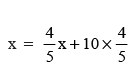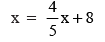Transposing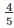x to LHS, we have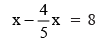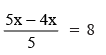x/5 = 8

Multiplying both sides by 5, we have
x = 8 × 5 = 40
∴ x = 40

8.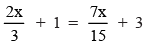Transposing 1 to RHS, we have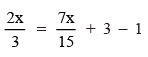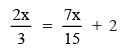Transposing 7x/15 to LHS, we have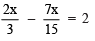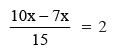3x/15 = 2

Multiplying both sides by 15, we have

3x = 2 × 15 = 30

Dividing both sides by 3, we have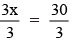∴ x = 10

9.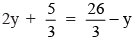Transposing 5/3  to RHS, we have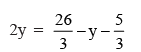Transposing (–y) to LHS, we have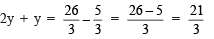3y = 7

Dividing both sides by 3, we have

3y = 3 = 7/3

∴ y = 7/3

10. 3m = 5m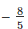Transposing 5m to LHS, we have

3m - 5m =-2m =Dividing both sides by (–2), we have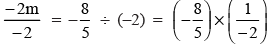∴ m = 4/5

Offer running on EduRev: Apply code STAYHOME200 to get INR 200 off on our premium plan EduRev Infinity!

93 docs|16 tests

,

,

,

,

,

,

,

,

,

,

,

,

,

,

,

,

,

,

,

,

,

;